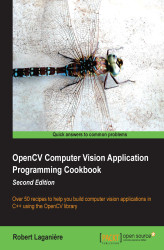•#### OpenCV Computer Vision Application Programming Cookbook#### Overview of this book

OpenCV Computer Vision Application Programming Cookbook Second EditionCreditswww.PacktPub.comPrefaceFree Chapter
Playing with ImagesManipulating PixelsProcessing Color Images with ClassesCounting the Pixels with HistogramsTransforming Images with Morphological OperationsFiltering the ImagesExtracting Lines, Contours, and ComponentsDetecting Interest PointsDescribing and Matching Interest PointsEstimating Projective Relations in ImagesProcessing Video SequencesIndex## Computing the Laplacian of an image

The Laplacian is another high-pass linear filter that is based on the computation of the image derivatives. As it will be explained, it computes second-order derivatives to measure the curvature of the image function.

### How to do it...

The OpenCV function, `cv::Laplacian`, computes the Laplacian of an image. It is very similar to the `cv::Sobel` function. In fact, it uses the same basic function, `cv::getDerivKernels`, in order to obtain its kernel matrix. The only difference is that there are no derivative order parameters since these ones are, by definition, second order derivatives.

For this operator, we will create a simple class that will encapsulate some useful operations related to the Laplacian. The basic methods are as follows:

```class LaplacianZC {

private:
// laplacian
cv::Mat laplace;
// Aperture size of the laplacian kernel
int aperture;

public:

LaplacianZC() : aperture(3) {}

// Set the aperture size of the kernel
...```